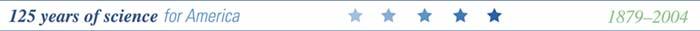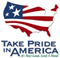#### By Charles Parrett and D.R. Johnson U.S. Geological Survey Water-Resources Investigations Report 03-4308

In cooperation with

Bureau of Indian Affairs, Bureau of Land Management,
Confederated Salish and Kootenai Tribes,
Montana Department of Natural Resources and Conservation,
Montana Department of Transportation, and the
U.S. Department of Agriculture Forest Service

### Abstract

Annual peak discharges having recurrence intervals of 2, 5, 10, 25, 50, 100, 200, and 500 years (T-year floods) were determined for 660 gaged sites in Montana and in adjacent areas of Idaho, Wyoming, and Canada, based on data through water year 1998. The updated flood-frequency information was subsequently used in regression analyses, either ordinary or generalized least squares, to develop equations relating T-year floods to various basin and climatic characteristics, equations relating T-year floods to active-channel width, and equations relating T-year floods to bankfull width. The equations can be used to estimate flood frequency at ungaged sites. Montana was divided into eight regions, within which flood characteristics were considered to be reasonably homogeneous, and the three sets of regression equations were developed for each region.

A measure of the overall reliability of the regression equations is the average standard error of prediction. The average standard errors of prediction for the equations based on basin and climatic characteristics ranged from 37.4 percent to 134.1 percent. Average standard errors of prediction for the equations based on active-channel width ranged from 57.2 percent to 141.3 percent. Average standard errors of prediction for the equations based on bankfull width ranged from 63.1 percent to 155.5 percent. In most regions, the equations based on basin and climatic characteristics generally had smaller average standard errors of prediction than equations based on active-channel or bankfull width. An exception was the Southeast Plains Region, where all equations based on active-channel width had smaller average standard errors of prediction than equations based on basin and climatic characteristics or bankfull width.

Methods for weighting estimates derived from the basin- and climatic-characteristic equations and the channel-width equations also were developed. The weights were based on the cross correlation of residuals from the different methods and the average standard errors of prediction. When all three methods were combined, the average standard errors of prediction ranged from 37.4 percent to 120.2 percent. Weighting of estimates reduced the standard errors of prediction for all T-year flood estimates in four regions, reduced the standard errors of prediction for some T-year flood estimates in two regions, and provided no reduction in average standard error of prediction in two regions. A computer program for solving the regression equations, weighting estimates, and determining reliability of individual estimates was developed and placed on the USGS Montana District World Wide Web page. A new regression method, termed Region of Influence regression, also was tested. Test results indicated that the Region of Influence method was not as reliable as the regional equations based on generalized least squares regression.

Two additional methods for estimating flood frequency at ungaged sites located on the same streams as gaged sites also are described. The first method, based on a drainage-area-ratio adjustment, is intended for use on streams where the ungaged site of interest is located near a gaged site. The second method, based on interpolation between gaged sites, is intended for use on streams that have two or more streamflow-gaging stations.

### Contents

Abstract
Introduction
Purpose and scope
Acknowledgements
General flood conditions
Methods for estimating flood frequency
Flood-frequency analysis
Flood-frequency data
Regional skew map
Mixed-population flood-frequency analysis
Regional flood-frequency relations
Basin-characteristics regression analysis
Channel-width regression analysis
Limitations of regression equations
Comparison of regional relations with those from previous study
Maximum recorded floods and envelope lines
Weighted regression estimates
Region of Influence regression
Estimating flood frequency on gaged streams
Ungaged sites on gaged streams
Weighted estimates at gaged sites
Example applications (examples 1, 2, and 3)
Summary
References cited
Appendix
Data

### Figures

1.  Map showing hydrologic regions and streamflow-gaging stations having compiled flood-frequency characteristics, Montana and adjacent areas in Idaho, Wyoming, and Canada (PLATE 1).
2.    Box plots showing variability in monthly occurrence of annual peak discharge by region, Montana.
3.    Graph showing example flood-frequency curve based on log-Pearson Type III probability distribution.
4.    Map showing regional skew coefficients for Montana.
5.    Graphs showing example flood-frequency curve based on mixed-population analysis.
6.    Map showing mean annual precipitation for the West and Northwest Regions, Montana, base period 1941-70 (PLATE 1).
7.    Diagrammatic sketch of a typical stream cross section showing active-channel and bankfull widths.
8.    Diagrammatic sketch of a typical alluvial stream showing best location for measuring channel width.
9.    Graphs showing comparison of new basin-characteristics equations with equations in previous U.S. Geological Survey report.
10.    Graphs showing maximum known floods, regional and national envelope lines, and regression lines relating 100-year flood to drainage area, West, Northwest, Northwest Foothills, and Northeast Plains Regions, Montana.
11.    Graphs showing maximum known floods, regional and national envelope lines, and regression lines relating 100-year flood to drainage area, East-Central Plains, Southeast Plains, Upper Yellowstone-Central Mountain, and Southwest Regions, Montana.
12.    Graphs showing average standard errors of prediction for various combinations of estimation methods in West, Northwest, Northwest Foothills, and Northeast Plains Regions, Montana.
13.    Graphs showing average standard errors of prediction for various combinations of estimation methods in East-Central Plains, Southeast Plains, Upper Yellowstone-Central Mountain, and Southwest Regions, Montana.

Tables

1.    Hydrologic regions and flood characteristics in Montana.
2.    Basin-characteristics, channel-width, and flood-frequency data for selected streamflow-gaging stations, Montana and adjacent areas Idaho, Wyoming, and Canada.
3.    State Map Errors for various methods of determining regional skew.
4.    Regression equations based on basin characteristics.
5.    Student's t  for confidence interval of 90 percent for different regions.
6.    Range of values of basin characteristics used to develop regression equations.
7.    Regression equations based on active-channel width.
8.    Regression equations based on bankfull width.
9.    Range of channel widths used to develop regression equations.
10.  Comparison of results for estimation of 100-year flood from new basin-characteristics equations with those from previous equations.
11.    Cross-correlation coefficients between residuals for combinations of different estimation methods.
12.    Weights and average standard errors of prediction for various combinations of estimation methods.
13.     Regression coefficients for ordinary least squares regressions relating T-year flood to drainage area.
14.    (XTX)-1 and (XT
L-1X)-1 matrices for regression equations based on basin characteristics.
15.    (XTX)-1and (XT
L-1X)-1 matrices for regression equations based on active-channel width.
16.    (XTX)-1 and (XT
L-1X)-1 matrices for regression equations based on bankfull width.

The full report is available in Adobe Acrobat® Portable Document Format (PDF).  Figure 1 and figure 6 are combined (PLATE 1) in a separate PDF document.  You will need Adobe Acrobat Reader®, version 5 or higher, to view or print the PDF documents.  If you do not already have this free viewing software or need to upgrade your version, download Adobe Acrobat Reader®.

 AccessibilityFOIAPrivacyPolicies and Notices U.S. Department of the Interior, U.S. Geological Survey Persistent URL: Page Contact Information: Contact USGS Last modified: Wednesday, December 07 2016, 01:28:38 PM